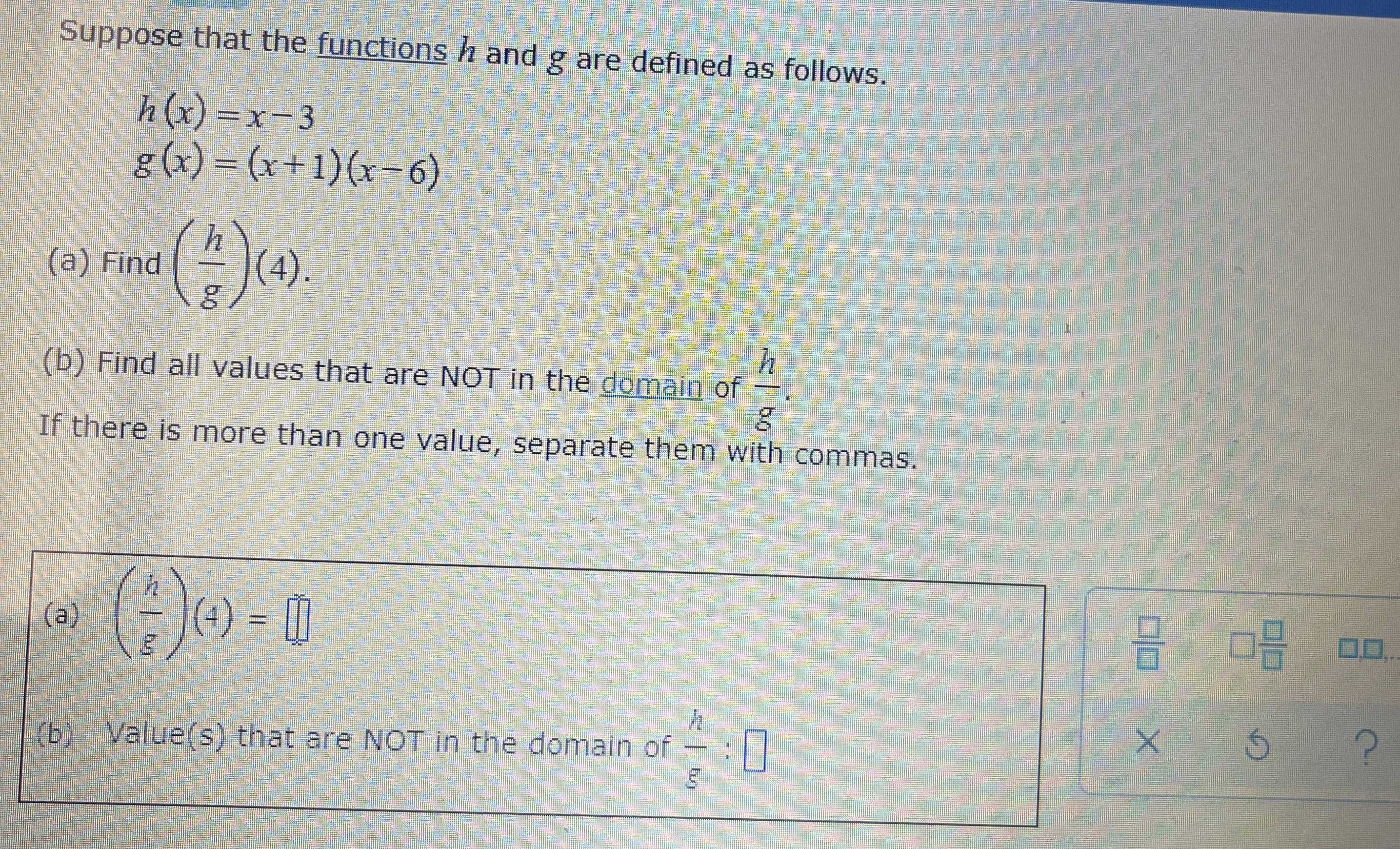### Still have math questions?

Algebra
QuestionSuppose that the functions $$h$$ and $$g$$ are defined as follows.

$$h ( x ) = x - 3$$

$$g ( x ) = ( x + 1 ) ( x - 6 )$$

(a) Find $$( \frac { h } { g } ) ( 4 )$$ .

(b) Find all values that are NOT in the domain of $$\frac { h } { g }$$

If there is more than one value, separate them with commas.

a) $$\frac{- 1}{10}$$